# 一、任务

%matplotlib inline
import numpy as np
import matplotlib.pyplot as plt
from scipy import stats

import seaborn as sns;sns.set() # 使用seaborn的默认设置

# 随机来点数据  make_blobs为聚类产生数据集
from sklearn.datasets.samples_generator import make_blobs

# center：产生数据的中心点，默认值3
X, y = make_blobs(n_samples=50, centers=2, random_state=0, cluster_std=0.60)
plt.scatter(X[:, 0], X[:, 1], c=y, s=50, cmap='autumn')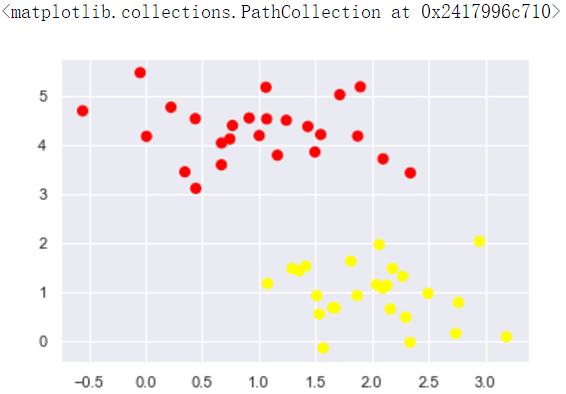xfit = np.linspace(-1, 3.5)
plt.scatter(X[:, 0], X[:, 1], c=y, s=50, cmap='autumn')
plt.plot([0.6], [2.1], 'x', color='red', markeredgewidth=2, markersize=10)

for m, b in [(1, 0.65), (0.5, 1.6), (-0.2, 2.9)]:
plt.plot(xfit, m * xfit + b, '-k')

plt.xlim(-1, 3.5)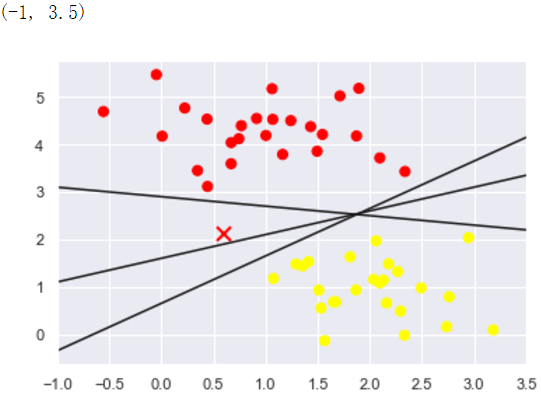xfit = np.linspace(-1, 3.5)
plt.scatter(X[:, 0], X[:, 1], c=y, s=50, cmap='autumn')

for m, b, d in [(1, 0.65, 0.33), (0.5, 1.6, 0.55), (-0.2, 2.9, 0.2)]:
yfit = m * xfit + b
plt.plot(xfit, yfit, '-k')
plt.fill_between(xfit, yfit - d, yfit + d, edgecolor='none',
color='#AAAAAA', alpha=0.4) # alpha透明度

plt.xlim(-1, 3.5);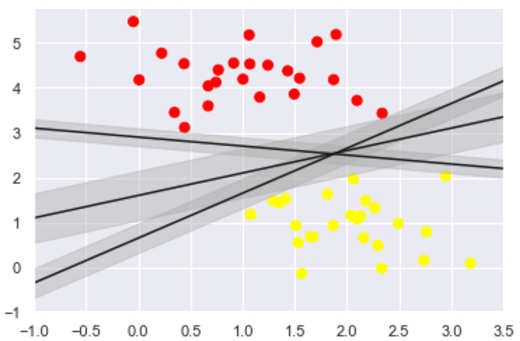# 二、训练一个基本的SVM

from sklearn.svm import SVC  # Support Vector Classifier
model = SVC(kernel='linear') # 线性核函数
model.fit(X, y)


SVC(C=1.0, cache_size=200, class_weight=None, coef0=0.0,
decision_function_shape='ovr', degree=3, gamma='auto_deprecated',
kernel='linear', max_iter=-1, probability=False, random_state=None,
shrinking=True, tol=0.001, verbose=False)

#绘图函数
def plot_svc_decision_function(model, ax=None, plot_support=True):
"""Plot the decision function for a 2D SVC"""
if ax is None:
ax = plt.gca() # get子图
xlim = ax.get_xlim()
ylim = ax.get_ylim()

# create grid to evaluate model
x = np.linspace(xlim, xlim, 30)
y = np.linspace(ylim, ylim, 30)
Y, X = np.meshgrid(y, x) # 生成网格点和坐标矩阵
xy = np.vstack([X.ravel(), Y.ravel()]).T     # 堆叠数组
P = model.decision_function(xy).reshape(X.shape)

# plot decision boundary and margins
ax.contour(X, Y, P, colors='k',
levels=[-1, 0, 1], alpha=0.5,
linestyles=['--', '-', '--']) # 生成等高线 - -

# plot support vectors
if plot_support:
ax.scatter(model.support_vectors_[:, 0],
model.support_vectors_[:, 1],
s=300, linewidth=1, facecolors='none');
ax.set_xlim(xlim)
ax.set_ylim(ylim)


plt.scatter(X[:, 0], X[:, 1], c=y, s=50, cmap='autumn')
plot_svc_decision_function(model);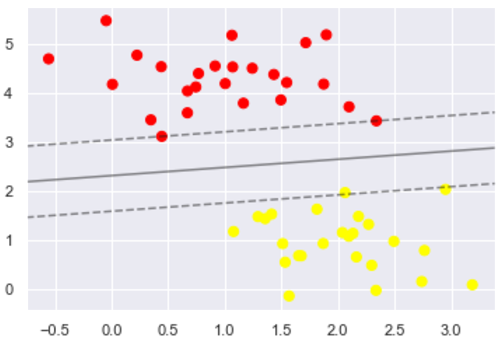model.support_vectors_


array([[0.44359863, 3.11530945],
[2.33812285, 3.43116792],
[2.06156753, 1.96918596]])

def plot_svm(N=10, ax=None):
X, y = make_blobs(n_samples=200, centers=2,
random_state=0, cluster_std=0.60)
X = X[:N]
y = y[:N]
model = SVC(kernel='linear', C=1E10)
model.fit(X, y)

ax = ax or plt.gca()
ax.scatter(X[:, 0], X[:, 1], c=y, s=50, cmap='autumn')
ax.set_xlim(-1, 4)
ax.set_ylim(-1, 6)
plot_svc_decision_function(model, ax)

fig, ax = plt.subplots(1, 2, figsize=(16, 6))
for axi, N in zip(ax, [60, 120]):
plot_svm(N, axi)
axi.set_title('N = {0}'.format(N))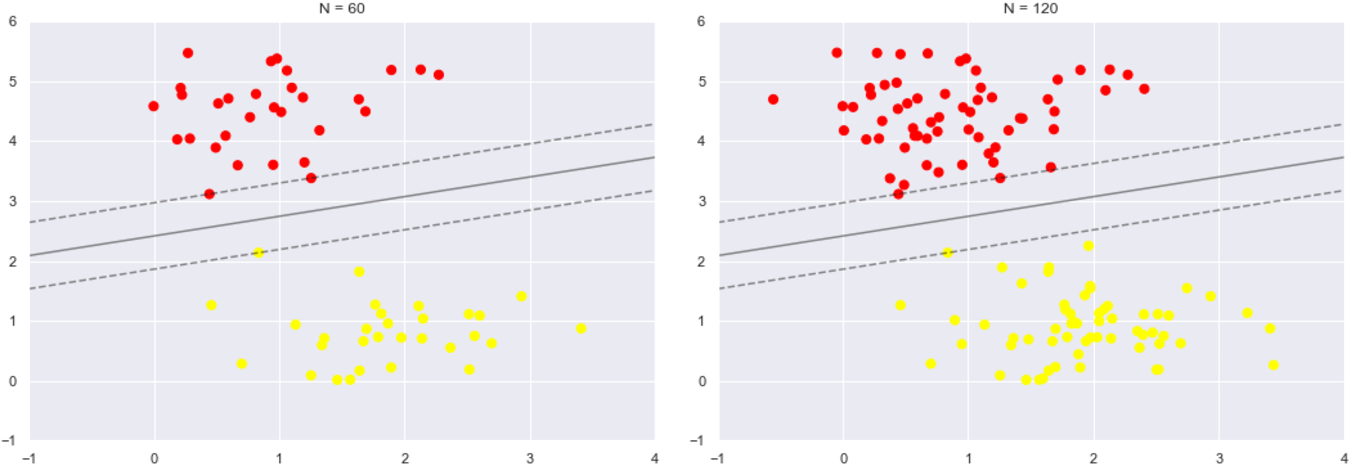# 三、引入核函数的SVM

from sklearn.datasets.samples_generator import make_circles
X, y = make_circles(100, factor=.1, noise=.1) # 二维圆形数据 factor 内外圆比例 (0,1)

clf = SVC(kernel='linear').fit(X, y)

plt.scatter(X[:, 0], X[:, 1], c=y, s=50, cmap='autumn')
plot_svc_decision_function(clf, plot_support=False);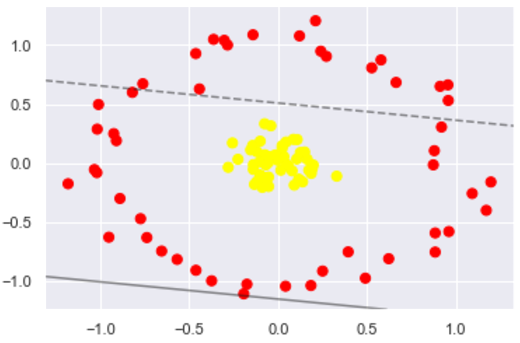#加入了新的维度r
from mpl_toolkits import mplot3d
r = np.exp(-(X ** 2).sum(1))
def plot_3D(elev=30, azim=30, X=X, y=y):
ax = plt.subplot(projection='3d')
ax.scatter3D(X[:, 0], X[:, 1], r, c=y, s=50, cmap='autumn')
ax.view_init(elev=elev, azim=azim) # 设置3D视图的角度  一般都为45
ax.set_xlabel('x')
ax.set_ylabel('y')
ax.set_zlabel('r')

plot_3D(elev=45, azim=45, X=X, y=y)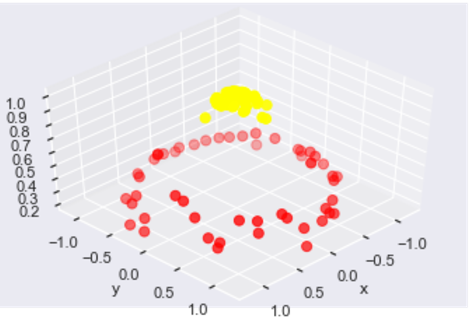#加入径向基函数
clf = SVC(kernel='rbf', C=1E6)
clf.fit(X, y)


SVC(C=1000000.0, cache_size=200, class_weight=None, coef0=0.0,
decision_function_shape='ovr', degree=3, gamma='auto_deprecated',
kernel='rbf', max_iter=-1, probability=False, random_state=None,
shrinking=True, tol=0.001, verbose=False)

#这回牛逼了！
plt.scatter(X[:, 0], X[:, 1], c=y, s=50, cmap='autumn')
plot_svc_decision_function(clf)
plt.scatter(clf.support_vectors_[:, 0], clf.support_vectors_[:, 1],
s=300, lw=1, facecolors='none');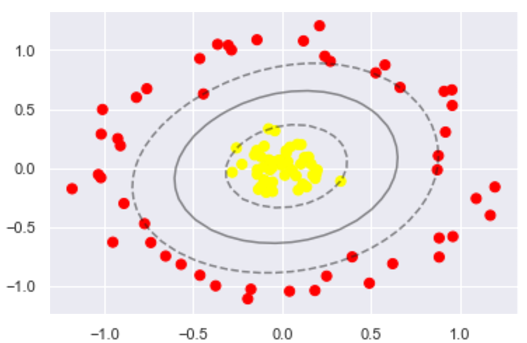# 四、软间隔问题

X, y = make_blobs(n_samples=100, centers=2,
random_state=0, cluster_std=0.8)
plt.scatter(X[:, 0], X[:, 1], c=y, s=50, cmap='autumn');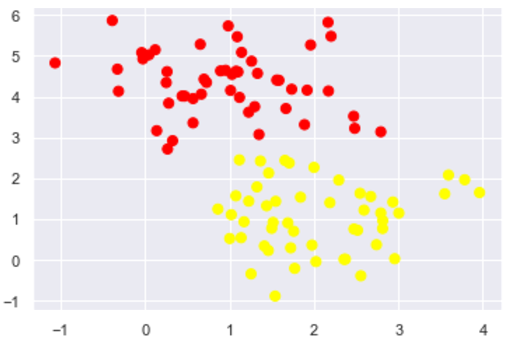X, y = make_blobs(n_samples=100, centers=2,
random_state=0, cluster_std=0.8)

fig, ax = plt.subplots(1, 2, figsize=(16, 6))

for axi, C in zip(ax, [10.0, 0.1]):
model = SVC(kernel='linear', C=C).fit(X, y)
axi.scatter(X[:, 0], X[:, 1], c=y, s=50, cmap='autumn')
plot_svc_decision_function(model, axi)
axi.scatter(model.support_vectors_[:, 0],
model.support_vectors_[:, 1],
s=300, lw=1, facecolors='none');
axi.set_title('C = {0:.1f}'.format(C), size=14)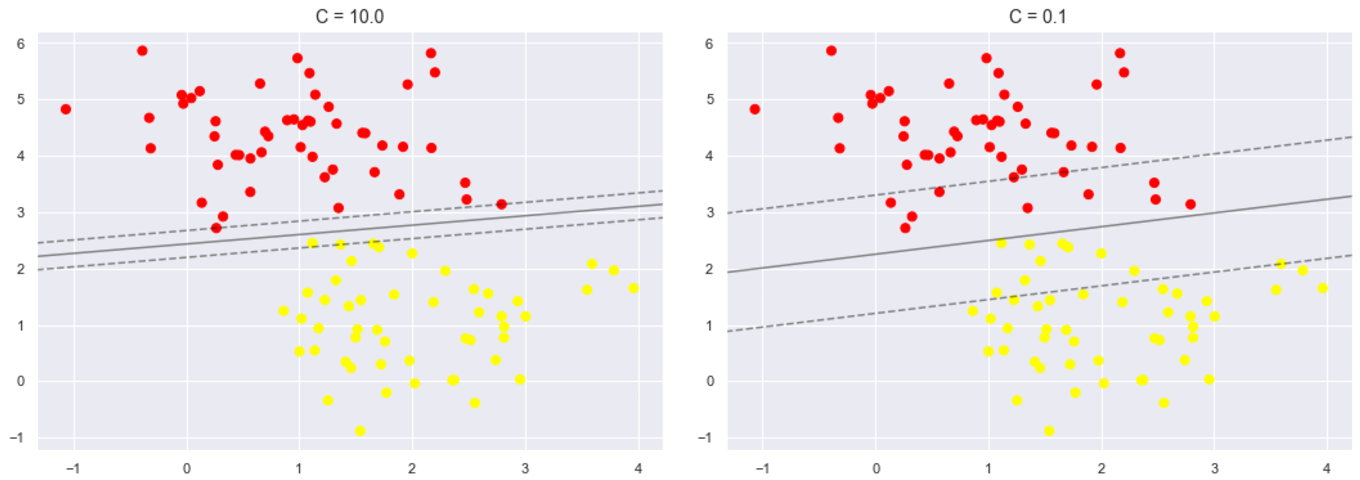X, y = make_blobs(n_samples=100, centers=2,
random_state=0, cluster_std=1.1)

fig, ax = plt.subplots(1, 2, figsize=(16, 6))

for axi, gamma in zip(ax, [10.0, 0.1]):
model = SVC(kernel='rbf', gamma=gamma).fit(X, y)
axi.scatter(X[:, 0], X[:, 1], c=y, s=50, cmap='autumn')
plot_svc_decision_function(model, axi)
axi.scatter(model.support_vectors_[:, 0],
model.support_vectors_[:, 1],
s=300, lw=1, facecolors='none');
axi.set_title('gamma = {0:.1f}'.format(gamma), size=14)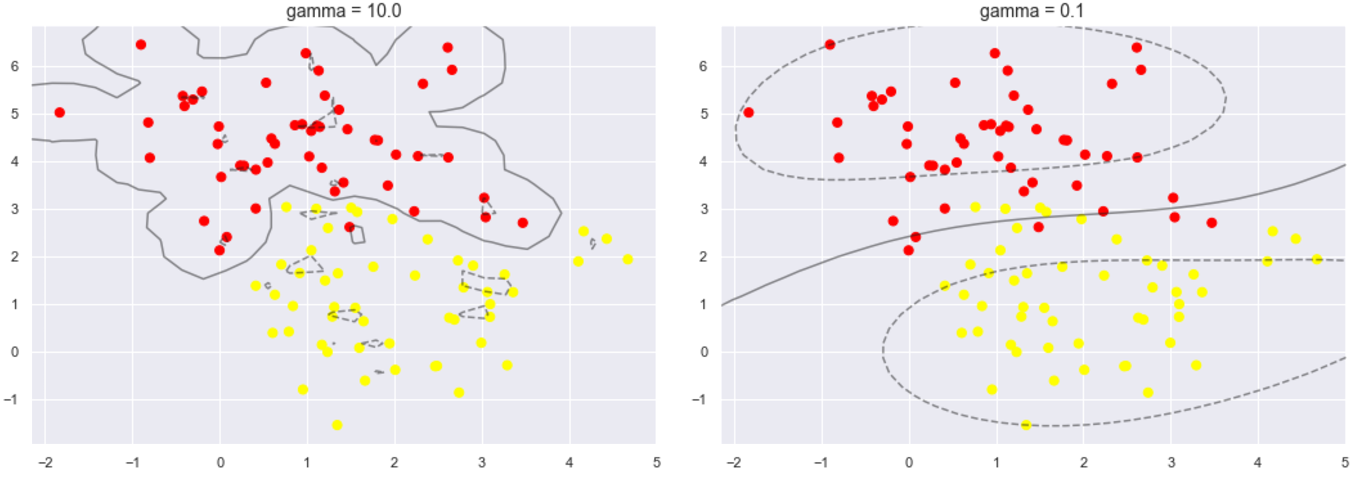# 四、总结

posted @ 2019-09-28 22:04 |旧市拾荒| 阅读(...) 评论(...) 编辑 收藏